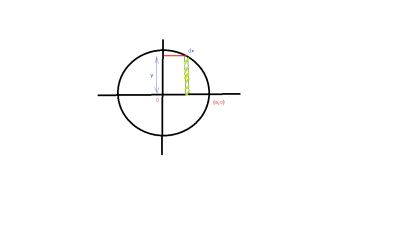# Use a line integral to find the area of the region R bounded by the graph of x^2+y^2=a^2.

## Question:

Use a line integral to find the area of the region {eq}R {/eq} bounded by the graph of {eq}x^2+y^2=a^2 {/eq}.

## Equation of circle

Standard equation of circle having (h,k) as the center and r radius is following

{eq}(x-h)^2+(y-k)^2=r^2 \\ {/eq}

and the equation of circle having origin as its center is

{eq}x^2+y^2=r^2 {/eq}

## Answer and Explanation:

Total area bounded by the circle is four times the area bounded in first quadrant ,so we find area bounded by circle in first quadrant using line integral

comparing the standard equation of circle from given ,we get

center of circle (0,0) and radius is a.Now we take a strip in first quadrant as shown in figure .

Area of strip will be ydx.

Total area of circle will be

{eq}A=4\int_{0}^{a}ydx {/eq}

Now y at a particular value of x will be

{eq}y=\sqrt{a^2-x^2} {/eq}

So,Area A is{eq}A=4\int _{0}^{a}\sqrt{a^2-x^2}dx \\ A=4[\frac{x}{2}\sqrt{a^2-x^2}+\frac{a^2}{2}\sin^{-1}\frac{x}{a} ] \Big|_{0}^{a} {/eq}

Now,after putting the limits, we get

{eq}A=4[0+\frac{a^2}{2}\sin^{-1}1-0-0] \\ A=4\frac{a^2\pi}{4} \\ A=\pi a^2 {/eq}